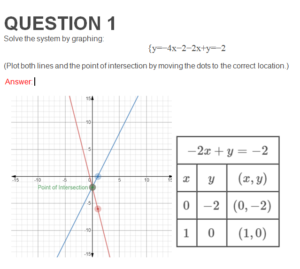# (Answered) MATH114N Week 5 Midterm Exam

QUESTION 1

Solve the system by graphing:

{y=−4x−2−2x+y=−2

(Plot both lines and the point of intersection by moving the dots to the correct location.)

QUESTION 2

Solve the following system of equations.

{y=−2x−92x+3y=−3

QUESTION 3

Solve the following system of equations:

{−2x−5y=−4

−3x−10y=−1

# ·

Translate the given phrase into an algebraic expression and simplify if possible: the quotient of 12 and the product of 3 and 4.

Note: Enter only the simplified result.

# QUESTION 5

The product of two positive consecutive integers is 72. Find the integers.

# QUESTION 6

Factor: 6a3−378a2−384a.

# QUESTION 7

Ozzie put 14gal of gas in his truck. Convert the volume to liters. Use 1qt=0.95L. Do not round your answer.

# QUESTION 8

Solve: 5(w+2.2)−4w=9.3.

# QUESTION 9

Steve drove for 812 hours at 72 miles per hour. How much distance did he travel?

# QUESTION 10

Graph the equation y=3x−3 by plotting points.

To plot points, click on a point on the graph and drag it to the desired location.

# QUESTION 11

Factor y2−42y−43.

# QUESTION 12

Solve: 12×2+17x+5=0.

(Use a comma to separate multiple solutions.)

# QUESTION 13

Solve: (11d+4)(9d+7)=0.

(Use a comma to separate multiple solutions.)

# QUESTION 14

Evaluate 2w2+8, when w=−4.

# QUESTION 15

Simplify: (12r3−6r2s)+(5rs2−10s3)−(7r2s−8rs2).

# QUESTION 17

Multiply: (−4p5)(53q4)

Note: Do not enter a mixed number, use an improper fraction.

# QUESTION 18

Factor 20c2+43c+14.

# QUESTION 19

Factor −6q3−66q2−60q.

# QUESTION 20

Simplify: z17z10.

# QUESTION 21

Factor: 38p3q3−10p.

# QUESTION 22

Factor cd+8d+5c+40 by grouping.

QUESTION 23

Solve: 5(s−1)+6=34.

• Enter an integer or a reduced fraction.

# QUESTION 24

Use the binomial squares pattern to multiply (5u3+4v)2.

# QUESTION 25

Multiply and collect like terms: (10m−1)(m−2).

# QUESTION 26

Factor: n2−8n+16.

# QUESTION 27

Find the quotient: (−81u6v6+18u5v5)÷(−9u2v4)

Factor: 64m2−25.

# QUESTION 29

Simplify: m14⋅mu.

Solution:

# QUESTION 30

Simplify: (23)4